﻿ 一种改进的非定常激波装配算法Download PDF文章快速检索 高级检索

1. 大连理工大学 航空航天学院, 大连 116024;
2. 中国人民解放军95791部队, 酒泉 735018

CHANG Siyuan1, BAI Xiaozheng1, CUI Xiaoqiang2, LIU Jun11. School of Aeronautics and Astronautics, Dalian University of Technology, Dalian 116024, China;
2. China PLA 95791 Unit, Jiuquan 735018, China
Abstract: Based on the unstructured dynamic grids technique and the cell-centered finite volume method, an improved unsteady shock-fitting algorithm is proposed to deal with unsteady flow containing moving shock waves. First, for the problem of shock wave propagating along the straight/curved wall, the motion models of wall discontinuity nodes are built respectively. Second, automatically distributing the discontinuity nodes on the basic of Bézier curve fitting method is realized to ensure fitted shock wave fronts move in a wide range without distortion. Then, by embedding the local module with automatic re-meshing, the efficiency and automation of the algorithm are significantly improved. Finally, for the motion of shock wave intersection point, a method of calculating velocity vector using displacement is designed to yield a fitting solution. Several simulation results show that the proposed algorithm can effectively deal with shock wave propagation problem. Compared with the shock-capturing method, the proposed method can extract much more flow field information and obtain a more straightforward and clearer map of flow field discontinuity.
Keywords: shock-fitting    unsteady    unstructured dynamic girds    finite volume method    computational fluid dynamics

2016年，Bonfiglioli等成功将其基于非结构网格和格点型有限体积法的装配算法拓展到非定常计算并模拟了激波与涡的相互作用，随后又进一步模拟了运动激波正规反射问题；刘君等模拟了运动激波与氦气柱的相互作用，采用装配方法对气柱界面进行装配，而运动激波仍用捕捉法进行模拟；随后，邹东阳等对等截面通道内的运动激波进行装配，与理论值比较考察了算法的计算精度；此外，作者所在团队采用边界装配策略对超高速弹丸发射时头部大范围运动的强激波进行处理，拓展了装配法在此类问题中的应用。然而，从以上研究来看，装配的激波只在直壁面上运动，而对于激波在弯曲壁面上的传播并未涉及；在整个计算中，装配的激波阵面大多不能自发地实现大变形，如拉伸、缩短和弯曲，往往需要人为调整改变装配激波点的分布；且当激波运动致使流场网格质量变差时，经常需要人为重新替换网格，算法的自动化程度比较低，计算代价比较大；最重要的一点，由于缺少成熟有效的基于非结构网格的运动激波探测(Shock Detection)算法，目前尚不能自动处理激波发生拓扑变化这类情况，如激波的正规反射向马赫反射演化、激波的新生与弱化等。

1 自适应间断装配求解器

1.1 激波捕捉算法

 $\left\{ \begin{matrix} \frac{\partial }{\partial t}\iiint\limits_{V}{\mathit{\boldsymbol{Q}}\text{d}\sigma \text{+}\mathop{{\int\!\!\!\!\!\int}\mkern-21mu \bigcirc}\limits_S \left( {{\mathit{\boldsymbol{F}}_c}}-\mathit{\boldsymbol{Q}}\cdot {{\mathit{\boldsymbol{x}}}_{\text{c}}} \right)\cdot \mathit{\boldsymbol{n}}\text{d}\psi =\mathrm{0} } \\ \mathit{\boldsymbol{Q}}={{\left[ \rho , \rho {{u}_{i}}, \rho E \right]}^{\text{T}}} \\ {{\mathit{\boldsymbol{F}}_c}}={{\left[ \rho {{u}_{i}}, \rho {{u}_{i}}{{u}_{j}}+p{{\delta }_{ij}}, \left( \rho E+p \right){{u}_{i}} \right]}^{\text{T}}} \\ E=\frac{P}{\rho \left( \gamma -1 \right)}+\frac{1}{2}{{u}_{i}}{{u}_{i}} \\ \end{matrix} \right.$ （1）

1.2 自适应间断装配算法 1.2.1 标记间断位置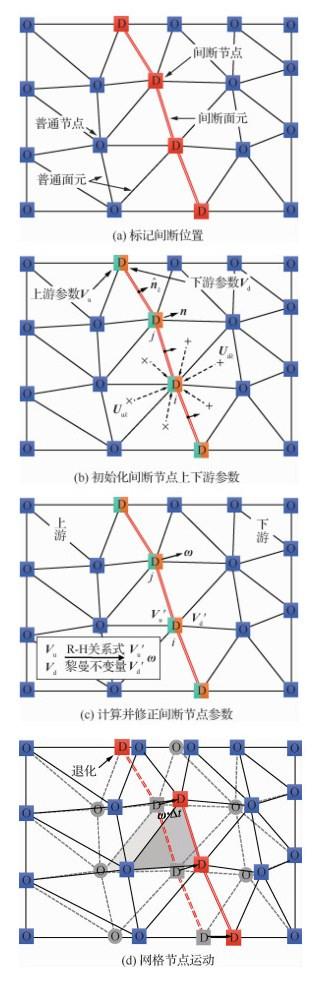图 1 自适应间断求解器的关键步骤 Fig. 1 Key procedures of ADFs
1.2.2 初始化间断节点参数

 ${\mathit{\boldsymbol{V}}_{u, i}} = \frac{{\sum\limits_{k = 1}^{{N_1}} {{\alpha _{ik}}{\mathit{\boldsymbol{U}}_{{\rm{u}}k}}} }}{{\sum\limits_{k = 1}^{{N_1}} {{\alpha _{ik}}} }}, {\mathit{\boldsymbol{V}}_{d, i}} = \frac{{\sum\limits_{k = 1}^{{N_2}} {{\alpha _{ik}}{\mathit{\boldsymbol{U}}_{{\rm{d}}k}}} }}{{\sum\limits_{k = 1}^{{N_2}} {{\alpha _{ik}}} }}$ （2）

 ${{\mathit{\boldsymbol{n}}}_{j}}=\frac{\sum\limits_{k=1}^{{{N}_{3}}}{\alpha _{jk}^{'}{{\widehat{\mathit{\boldsymbol{n}}}}_{k}}}}{\sum\limits_{k=1}^{{{N}_{3}}}{\alpha _{jk}^{'}}}$ （3）

1.2.3 计算并修正间断节点参数

1.2.2节得到的间断节点两侧参数并不满足描述间断的跳跃关系式，如Rankine-Hugoniot(R-H)关系式。对激波来讲，在激波坐标系下，由于上游流动是超声速的，即下游流动信息理论上不会污染上游参数，因此上游流动参数Vu无需修正。沿着间断节点法向n，一共有4个参数需要计算，即修正后的激波下游流动参数Vd=[ρ′d, u′d, p′d]和激波节点的运动速度ω=ω·n。R-H关系式和下游特征相容关系式分别为

 \left\{ \begin{matrix} \rho {{'}_{\text{d}}}\left( u_{\text{d}}^{'}-\omega \right)={{\rho }_{\text{u}}}\left( {{u}_{\text{u}}}-\omega \right) \\ \rho {{'}_{\text{d}}}{{\left( u_{\text{d}}^{\text{ }\!\!'\!\!\text{ }}-\omega \right)}^{2}}+p_{\text{d}}^{'}={{\rho }_{\text{u}}}{{\left( {{u}_{\text{u}}}-\omega \right)}^{2}}+{{p}_{\text{u}}} \\ \begin{align} & \frac{\gamma }{\gamma -1}\cdot \frac{p_{\text{d}}^{'}}{\rho _{\text{d}}^{\text{ }\!\!'\!\!\text{ }}}+\frac{{{\left( u_{\text{d}}^{\text{ }\!\!'\!\!\text{ }}-\omega \right)}^{2}}}{2}= \\ & \frac{\gamma }{\gamma -1}\cdot \frac{{{p}_{\text{u}}}}{{{\rho }_{\text{u}}}}+\frac{{{\left( {{u}_{u}}-\omega \right)}^{2}}}{2} \\ \end{align} \\ \end{matrix} \right. （4）
 $\frac{2}{\gamma -1}\sqrt{\gamma p_{\text{d}}^{'}/\rho _{\text{d}}^{'}}-u_{\text{d}}^{\text{ }\!\!'\!\!\text{ }}=\frac{2}{\gamma -1}\sqrt{\gamma {{p}_{\text{d}}}/{{\rho }_{\text{d}}}}-{{u}_{\text{d}}}$ （5）

 $Ma_{\text{u}}^{\text{ref}}=\frac{{{u}_{\text{u}}}-\omega }{\sqrt{\gamma {{p}_{\text{u}}}/{{\rho }_{\text{u}}}}}$ （6）

1.2.4 网格节点运动

 $\mathit{\boldsymbol{X}}_j^{t + \Delta t} = \mathit{\boldsymbol{X}}_j^t + {\mathit{\boldsymbol{\omega }}_j}\Delta t$ （7）

1.2.5 流场更新

 $\mathit{\boldsymbol{F}}_j^D = {\left[ {\begin{array}{*{20}{c}} \phi \\ {\phi {u_i} + p{A_i}}\\ {\phi E{\rm{ + }}p\mathit{\boldsymbol{u}} \cdot \mathit{\boldsymbol{A}}} \end{array}} \right]_{{\rm{u, }}j}}$ （8）

1.3 非定常激波装配的一些改进

1.3.1 间断节点沿壁面运动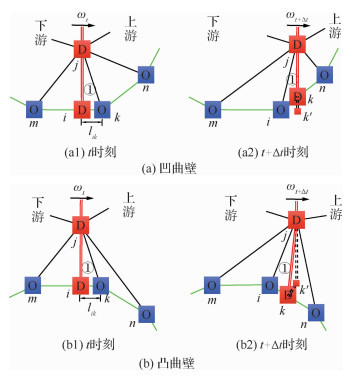图 2 间断节点沿曲壁面的运动 Fig. 2 Movements of discontinuity nodes along curved wall

1.3.2 间断节点分布的自动重构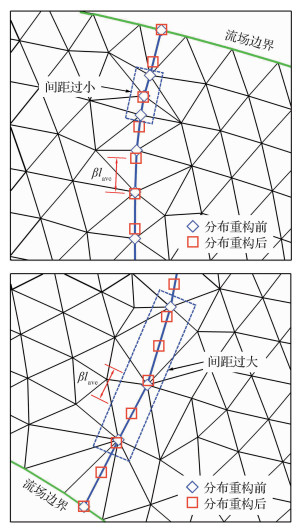图 3 间断节点分布重构前后的网格 Fig. 3 Grids before and after redistribution of discontinuity nodes

 ${l_k}/{l_{{\rm{ave}}}} < {\delta _{\min }}或{l_k}/{l_{{\rm{ave}}}} > {\delta _{\max }}$ （9）

1.3.3 流场网格的局部自动重构

1) 采用局部网格变形/重构策略能显著提高计算效率，同时重构单元数量的缩减很大程度上可以减小流场信息插值引入的误差。如图 4(a)所示，以间断位置作为起点向两侧搜寻网格，标记距离间断最近的n层网格单元作为可变形单元且可以重构，其他网格静止且不参与重构，即流场可分为“动网格区”(图 4阴影区)和“静网格区”。当激波在直壁面上运动时，n通常取10；而在曲壁面上运动时，由于网格重构较为频繁，动网格区可以取小一点，如n=3。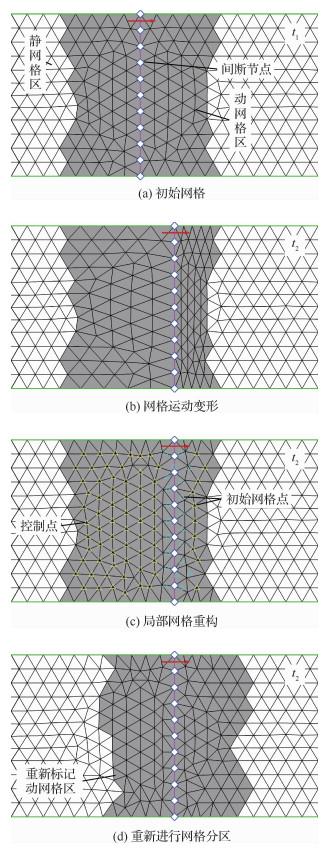图 4 间断附近局部网格自动重构(n=5) Fig. 4 Automatically local re-meshing around discontinuity front(n=5)

2) 当激波运动使得网格质量较差时(如图 4(b))，需要进行网格重构。考虑到计算初始网格通常用专业网格生成软件划分，网格质量可以控制得比较好(如大部分为正三角形)，因此ADFs在网格重构时利用了初始网格信息。如图 4(c)所示，提取位于重构区内的初始网格点，作为网格生成“控制点”供Triangle程序调用生成Delaunay三角形网格。注意，为了保证网格质量，距离间断过近(约一个网格尺寸内)的初始网格点不作为控制点。这样，经控制生成的大部分网格单元的尺寸和形状与初始高质量网格保持一致(对比图 4(a)图 4(c))，一定程度上减弱了网格重构对流场网格分布和拓扑的影响。

3) 每次完成网格重构后，由于旧网格变形区已经不太适合新的间断位置，因此需要重新标记动网格区和静网格区，如图 4(d)所示，即计算过程中流场网格重构区是动态变化的，随间断移动需要动态调整。

2 验证算例

2.1 激波绕射90°拐角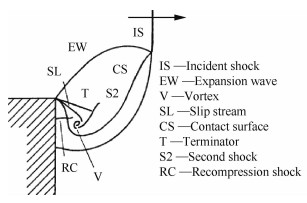图 5 强激波绕射90°拐角流场结构 Fig. 5 Flow field of strong shock wave diffraction at a 90° convex edge

 参数 入射激波马赫数Mas 激波下游流场马赫数 激波上、下游压强比 激波上、下游密度比 数值 2.4 1.157 6.553 3.212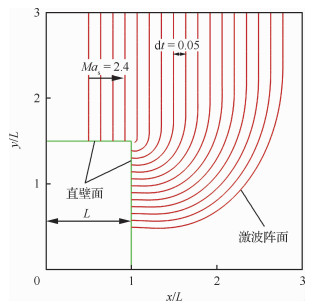图 6 不同时刻下绕射激波的装配阵面 Fig. 6 Fitted fronts of diffracted shock waves at various moments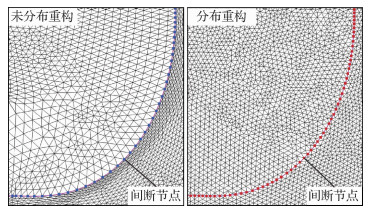图 7 算法改进前后的装配激波附近流场网格对比 Fig. 7 Comparison of flow field grids near fitted shock wave before and after algorithm improvement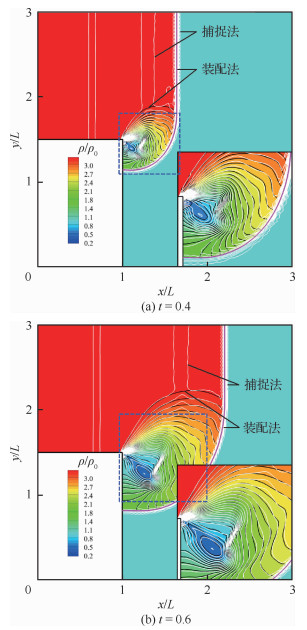图 8 不同时刻流场密度云图对比 Fig. 8 Comparison of flow field density contours at various moments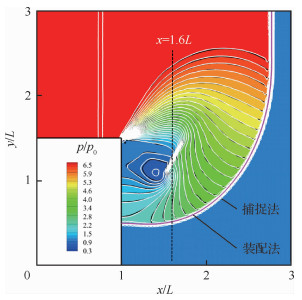图 9 t=0.8时刻流场压强云图对比 Fig. 9 Comparison of flow field pressure contours at t=0.8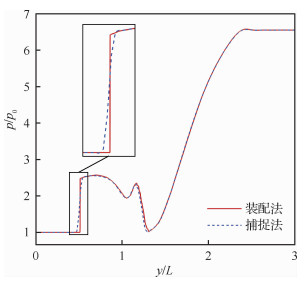图 10 x=1.6L处压强分布对比 Fig. 10 Comparison of pressure distributions at x=1.6L
2.2 二维激波管内激波增强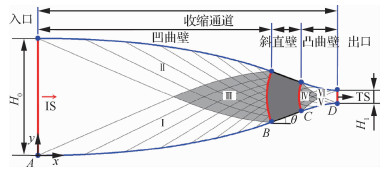图 11 收缩壁面型线设计示意图 Fig. 11 Sketch of contraction wall profile design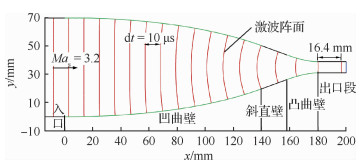图 12 激波管内不同时刻装配激波阵面 Fig. 12 Fitted shock wave fronts at various moments in shock tube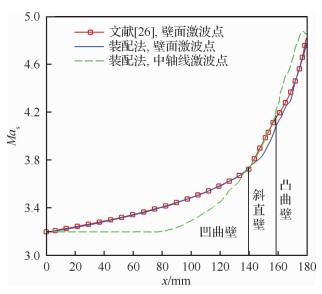图 13 激波在不同水平位置的强度对比 Fig. 13 Comparison of shock wave intensity at various horizontal locations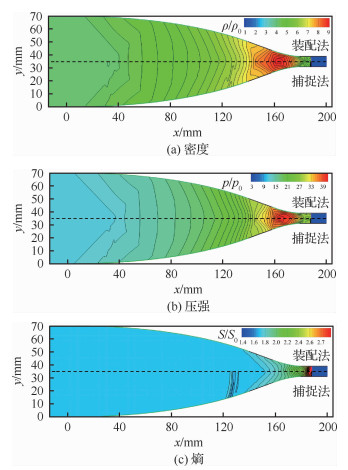图 14 t=165 μs时刻装配法和捕捉法模拟的流场对比 Fig. 14 Comparison of flow fields simulated by S-F and S-C at t=165 μs
2.3 弯管内激波传播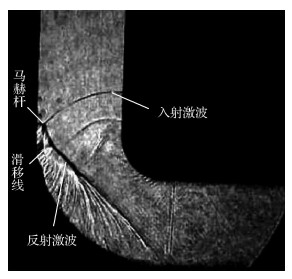图 15 激波在弯管中传播的试验阴影图 Fig. 15 Experimental shadow diagram of diffracted shock wave in curved tube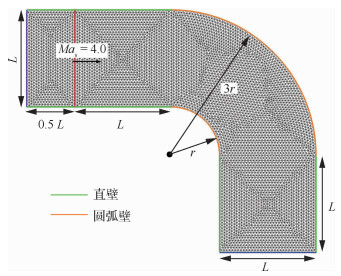图 16 计算区域、初始条件和网格 Fig. 16 Computational domain, initial condition and meshes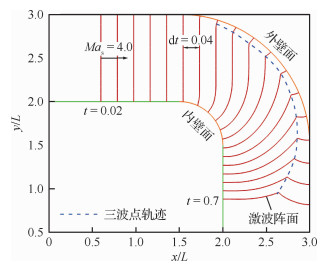图 17 不同时刻下L形弯管内装配的激波阵面 Fig. 17 Fitted shock wave fronts at various moments in L-shaped tube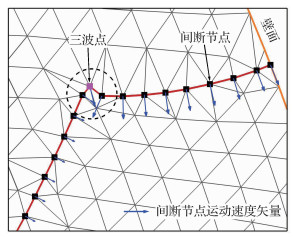图 18 未修正的三波点运动 Fig. 18 Motion of triple point before correction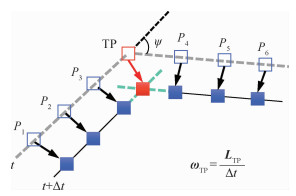图 19 三波点运动速度计算模型 Fig. 19 Calculation model of triple point motion speed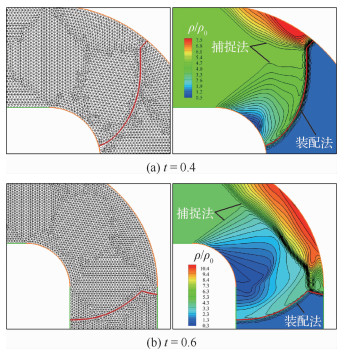图 20 不同时刻流场密度云图及网格对比 Fig. 20 Comparison of flow field density contours and meshes at various moments
3 结论

1) 当激波在曲壁面上运动时，运动间断节点可以在固定壁面网格节点的前提下实现装配。

2) 针对激波产生大变形、大位移运动问题，通过间断节点分布的自动重构保证激波阵面不失真，同时采用局部网格自动重构策略确保了网格的高质量，并提高了程序计算效率。

3) 对于激波相交点的运动，设计了一种根据位移推算速度的方法进行装配，数值算例表明该方法行之有效。

  CASPER J, CARPENTER M H. Computational considerations for the simulation of shock-induced sound[J]. SIAM Journal on Scientific Computing, 1998, 19(3): 813-828. Click to display the text  ARORA M, ROE P L. On postshock oscillations due to shock capturing schemes in unsteady flows[J]. Journal of Computational Physics, 1997, 130(1): 25-40. Click to display the text  ZAIDE D, ROE P L. Shock capturing anomalies and the jump conditions in one dimension: AIAA-2011-3686[R]. Reston, VA: AIAA, 2011.  PIROZZOLI S. Numerical methods for high-speed flows[J]. Annual Review of Fluid Mechanics, 2011, 43: 163-194. Click to display the text  JOHNSEN E, LARSSON J, BHAGATWALA A V, et al. Assessment of high-resolution methods for numerical simulations of compressible turbulence with shock waves[J]. Journal of Computational Physics, 2010, 229(4): 1213-1237. Click to display the text  MORETTI G. Computation of flows with shocks[J]. Annual Review of Fluid Mechanics, 1987, 19(1): 313-337. Click to display the text  NASUTI F, ONOFRI M. Analysis of unsteady supersonic viscous flows by a shock-fitting technique[J]. AIAA Journal, 1996, 34(7): 1428-1434. Click to display the text  SALAS M D. A shock-fitting primer[M]. Boca Raton, FL: CRC Press, 2009: 115-124.  PACIORRI R, BONFIGLIOLI A. A shock-fitting technique for 2D unstructured grids[J]. Computers & Fluids, 2009, 38(3): 715-726. Click to display the text  BONFIGLIOLI A, GROTTADAUREA M, PACIORRI R, et al. An unstructured, three-dimensional, shock-fitting solver for hypersonic flows[J]. Computers & Fluids, 2013, 73: 162-174. Click to display the text  ZOU D Y, XU C G, DONG H B, et al. A shock-fitting technique for cell-centered finite volume methods on unstructured dynamic meshes[J]. Journal of Computational Physics, 2017, 345: 866-882. Click to display the text  BONFIGLIOLI A, PACIORRI R, CAMPOLI L. Unsteady shock-fitting for unstructured grids[J]. International Journal for Numerical Methods in Fluids, 2016, 81(4): 245-261. Click to display the text  BONFIGLIOLI A, PACIORRI R, CAMPOLI L, et al. Development of an unsteady shock-fitting technique for unstructured grids[C]//30th International Symposium on Shock Waves 2. Berlin: Springer, 2017: 1501-1504.  刘君, 邹东阳, 董海波. 基于非结构变形网格的间断装配法原理[J]. 气体物理, 2017, 2(1): 13-20. LIU J, ZOU D Y, DONG H B. Principle of new discontinuity fitting technique based on unstructured moving grid[J]. Physics of Gases, 2017, 2(1): 13-20. (in Chinese) Cited By in Cnki | Click to display the text  邹东阳, 刘君, 邹丽. 可压缩流动激波装配在格心型有限体积法中的应用[J]. 航空学报, 2017, 38(11): 121363. ZOU D Y, LIU J, ZOU L. Applications of shock-fitting technique for compressible flow in cell-centered finite volume methods[J]. Acta Aeronautica et Astronautica Sinica, 2017, 38(11): 121363. (in Chinese) Cited By in Cnki | Click to display the text  常思源, 邹东阳, 刘君. 自适应间断装配法模拟弹道靶中超高速弹丸发射[J]. 实验流体力学, 2019, 33(2): 23-29. CHANG S Y, ZOU D Y, LIU J. Simulating hypersonic projectile launching process in the ballistic range by adaptive discontinuity fitting solver technique[J]. Journal of Experiments in Fluid Mechanics, 2019, 33(2): 23-29. (in Chinese) Cited By in Cnki | Click to display the text  CHANG S Y, BAI X Z, ZOU D Y, et al. An adaptive discontinuity fitting technique on unstructured dynamic grids[J]. Shock Waves, 2019, 29: 1103-1115. Click to display the text  刘君, 徐春光, 白晓征. 有限体积法和非结构动网格[M]. 北京: 科学出版社, 2016: 42-163. LIU J, XU C G, BAI X Z. Finite volume methods and unstructured dynamic grids technique[M]. Beijing: Science Press, 2016: 42-163. (in Chinese)  徐春光, 董海波, 刘君. 基于单元相交的混合网格精确守恒插值方法[J]. 爆炸与冲击, 2016, 36(3): 305-312. XU C G, DONG H B, LIU J. An accurate conservative interpolation method for the mixed grid based on the intersection of grid cells[J]. Explosion and Shock Waves, 2016, 36(3): 305-312. (in Chinese) Cited By in Cnki (1) | Click to display the text  BÉZIER P. Numerical control:Mathematics and applications[M]. London: Wiley, 1972.  SHEWCHUK J R. Triangle: Engineering a 2D quality mesh generator and Delaunay triangulator[C]//Workshop on Applied Computational Geometry. Berlin: Springer, 1996: 203-222.  KLEINE H, RITZERFELD E, GRÖNIG H. Shock wave diffraction-New aspects of an old problem[M]//Shock waves@Marseille Ⅳ. Berlin: Springer, 1995: 117-122.  HILLIER R. Computation of shock wave diffraction at a ninety degrees convex edge[J]. Shock Waves, 1991, 1(2): 89-98. Click to display the text  RIPLEY R C, LIEN F S, YOVANOVICH M M. Numerical simulation of shock diffraction on unstructured meshes[J]. Computers & Fluids, 2006, 35(10): 1420-1431. Click to display the text  詹东文, 杨剑挺, 杨基明, 等. 一种形状可控的激波增强管道型线设计新方法[J]. 航空学报, 2016, 37(8): 2408-2416. ZHAN D W, YANG J T, YANG J M, et al. A new method of wall profile design for shape-controllable shock wave enhancement[J]. Acta Aeronautica et Astronautica Sinica, 2016, 37(8): 2408-2416. (in Chinese) Cited By in Cnki | Click to display the text  詹东文.一种激波增强管壁型线设计方法[D].合肥: 中国科学技术大学, 2018: 48. ZHAN D W. An investigation of the channel profile design for shock wave enhancement[D]. Hefei: University of Science and Technology of China, 2018: 48(in Chinese).  袁雪强.弯曲爆震管中爆震传播特性及弯曲管道射流起爆机理研究[D].长沙: 国防科技大学, 2015: 29-30. YUAN X Q. Characteristics of detonation wave propagation and detonation initiation via hot jet in bend tubes[D]. Changsha: National University of Defense Technology, 2015: 29-30(in Chinese).
http://dx.doi.org/10.7527/S1000-6893.2019.23498

0

#### 文章信息

CHANG Siyuan, BAI Xiaozheng, CUI Xiaoqiang, LIU Jun

Acta Aeronautica et Astronautica Sinica, 2020, 41(2): 123498.
http://dx.doi.org/10.7527/S1000-6893.2019.23498